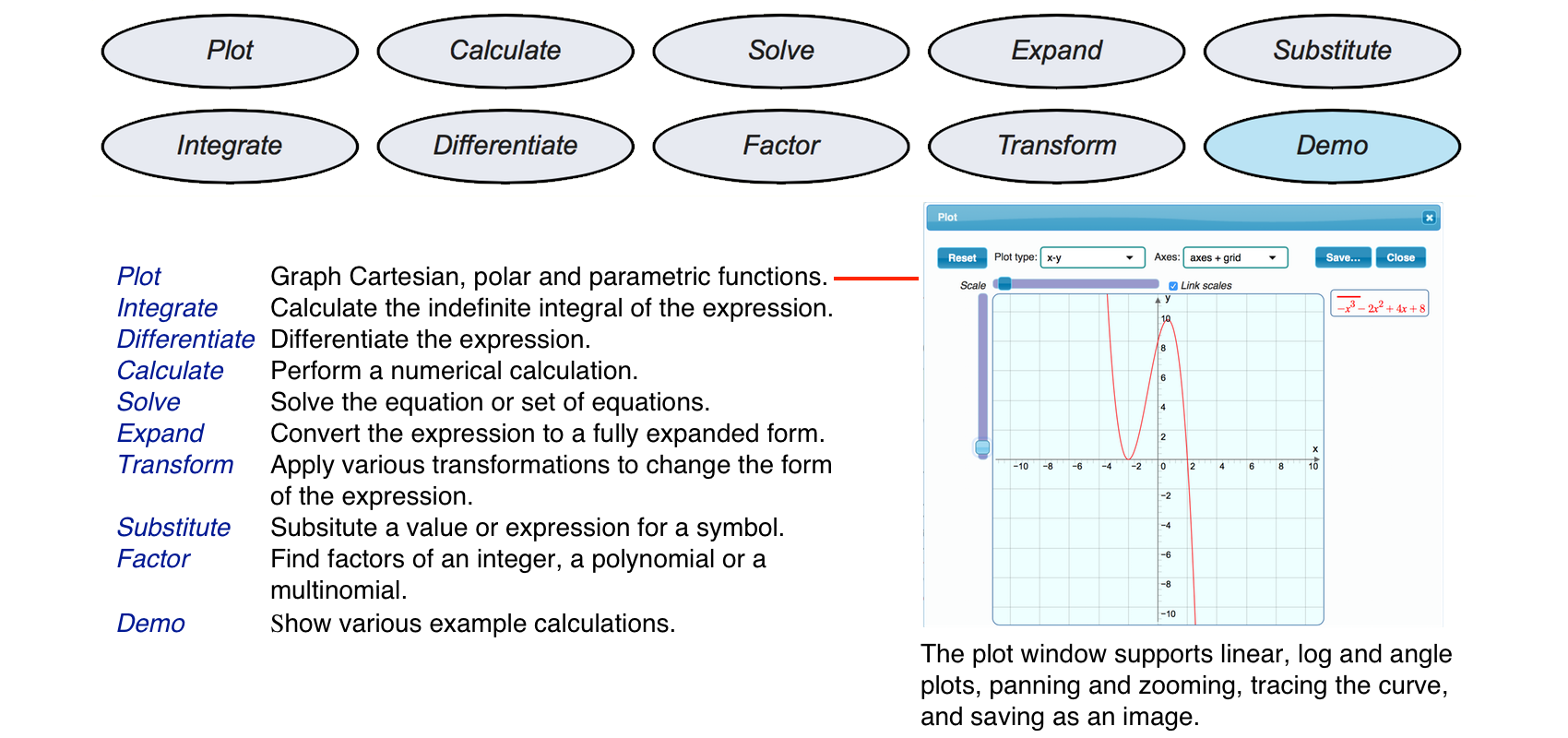BETA3Symbolic and numeric calculation

Entering Mathematical ExpressionsUsing the ButtonsAvailable Mathematical ActionsPlot Calculate Solve Expand Substitute Integrate Differentiate Factor Transform Demo